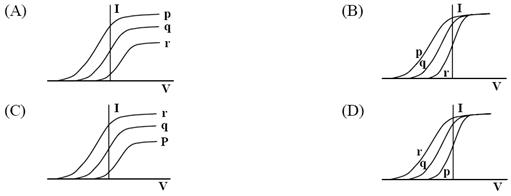Click to Chat

1800-1023-196

+91-120-4616500

CART 0

• 0

MY CART (5)

Use Coupon: CART20 and get 20% off on all online Study Material

ITEM
DETAILS
MRP
DISCOUNT
FINAL PRICE
Total Price: Rs.

There are no items in this cart.
Continue Shopping• Complete JEE Main/Advanced Course and Test Series
• OFFERED PRICE: Rs. 15,900
• View Details

```IIT JEE 2009 Physics Paper2 Code 1 Solutions

SINGLE CORRECT CHOICE TYPE

1.     Photoelectric effect experiments are performed using three different metal plates p, q and r having work functions fP = 2.0 eV, fq = 2.5 eV, and fr = 3.0 eV, respectively. A light beam containing wavelengths of 550 nm, 450 nm and 350 nm with equal intensities illuminates each of the plates. The correct 1-V graph for the experiment isSol.   (A)

VB = (1/e)[(hc/l) - f]

VP = (1/e)[(1240/550) - 2]eV  = 0.2545 V

Vq = (1/e)[(1240/450) - 2.5]eV  = 0.255 V

Vr = (1/e)[(1240/350) - 3]eV  = 0.5428 V

If n is the number of photons in unit time then nhc/l = I

=> iP : iq : ir = nP : nq = λP : λq : λT

2.     A uniform rod of length L and mass M is pivoted at the centre. Its two ends are attached to two springs of equal spring constants k. The springs are fixed to rigid supports as shown in the figure, and rod is free to oscillate in the horizontal plane. The rod is gently pushed through a small angle q in one direction and released. The frequency of oscillation is(A) 1/2π √(2k/M)

(B) 1/2π √(k/M)

(C) 1/2π √(6k/M)

(D) 1/2π √(24k/M)

Sol.   (C)

We have ,Restoring torque = –2 × k (l/2 θ ) l/2=(Id2 θ )/ dt2

(d2 θ )/ dt2 =(kl2/2 (-θ ))/(Ml2/12)

=> ω = √(6k/M)

Hence, frequency of oscillation is C

<< Back || Next >>
```### Course Features

• 731 Video Lectures
• Revision Notes
• Previous Year Papers
• Mind Map
• Study Planner
• NCERT Solutions
• Discussion Forum
• Test paper with Video Solution